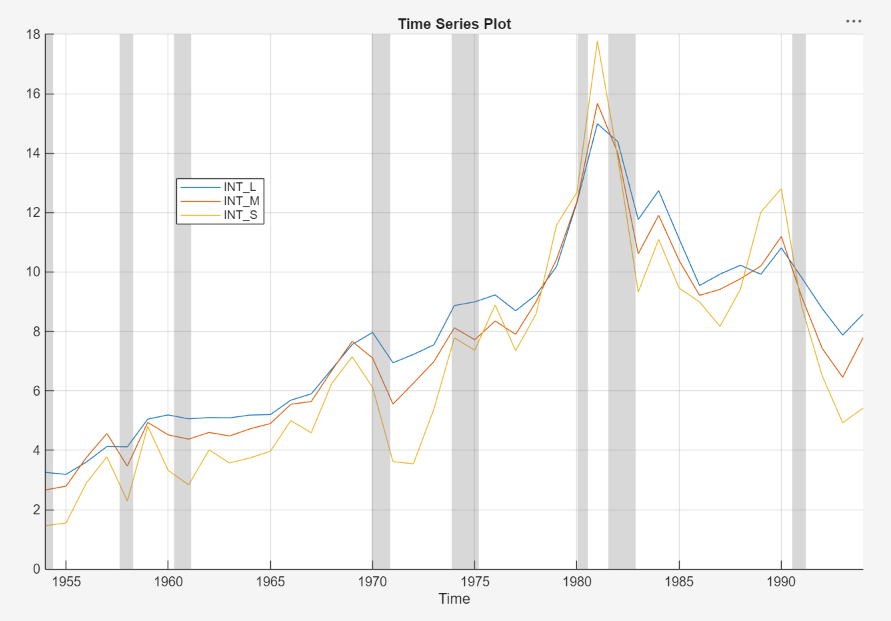## Plot Time Series Data Using Econometric Modeler App

These examples show how to plot univariate and multivariate time series data by using the Econometric Modeler app. After plotting time series, you can interact with the plots.

### Plot Univariate Time Series Data

This example shows how to plot univariate time series data, then overlay recession bands in the plot. The data set contains the quarterly US gross domestic product (GDP) prices from 1947 through 2005.

At the command line, load the `Data_GDP.mat` data set.

```load Data_GDP ```

`DataTimeTable` is a timetable containing time series data.

At the command line, open the Econometric Modeler app.

`econometricModeler`

Alternatively, open the app from the apps gallery (see Econometric Modeler).

Import `DataTimeTable` into the app:

1. On the Econometric Modeler tab, in the Import section, click the button.

2. In the Import Data dialog box, in the Import? column, select the check box for the `DataTimeTable` variable.

3. Click .

The variable `GDP` appears in the Time Series pane, and its time series plot appears in the Time Series Plot(GDP) figure window.

Overlay recession bands by right-clicking the plot and figure window, then selecting Show Recessions.

Overlay a grid by pausing on the plot and clicking.

Focus on the GDP from 1970 to the end of the sampling period:

1. Pause on the plot, then click.

2. Position the cross hair at (1970,12000), then drag the cross hair to (2005,3500).The GDP appears flat or decreasing before and during periods of recession.

### Plot Multivariate Time Series and Correlations

This example shows how to plot multiple series on the same time series plot, interact with the resulting plot, and plot the correlations among the variables. The data set, stored in `Data_Canada`, contains annual Canadian inflation and interest rates from 1954 through 1994.

At the command line, load the `Data_Canada.mat` data set.

`load Data_Canada`

At the command line, open the Econometric Modeler app.

`econometricModeler`

Alternatively, open the app from the apps gallery (see Econometric Modeler).

Import `DataTimeTable` into the app:

1. On the Econometric Modeler tab, in the Import section, click the button.

2. In the Import Data dialog box, in the Import? column, select the check box for the `DataTimeTable` variable.

3. Click .

The Canadian interest and inflation rate variables appear in the Time Series pane, and a time series plot containing all the series appears in the Time Series Plot(INF_C) figure window.

Overlay recession bands by right-clicking the plot and selecting Show Recessions.

Overlay a grid by pausing on the plot and clicking.

Remove the inflation rates (`INF_C` and `INF_G`) from the time series plot:

1. Right-click the plot.

2. Point to Show Time Series, then clear INF_C.

3. Repeat steps 1 and 2, but clear INF_G instead.Generate a correlation plot for all variables:

1. Select all variables in the Time Series pane.

2. Click the Plots tab, then click .

A correlations plot appears in the Correlations(INF_C) figure window.

Remove the inflation rate based on GDP (`INF_G`) from the correlations plot:

1. Right-click the plot.

2. Point to Show Time Series, then clear INF_G.All variables appear to be skewed to the right. According to the Pearson correlation coefficients (top-left of the off-diagonal plots):

• The inflation rate explains at least 70% of the variability in the interest rates (when used as a predictor in a linear regression).

• The interest rates are highly correlated; each explains at least 94% of the variability in another series.